# PHP为什么empty可以访问不存在的索引

``\$arr = []; echo 'empty: ', PHP_EOL; var_dump(empty(\$arr['1'])); echo 'is_array: ', PHP_EOL; var_dump(is_array(\$arr['1']));``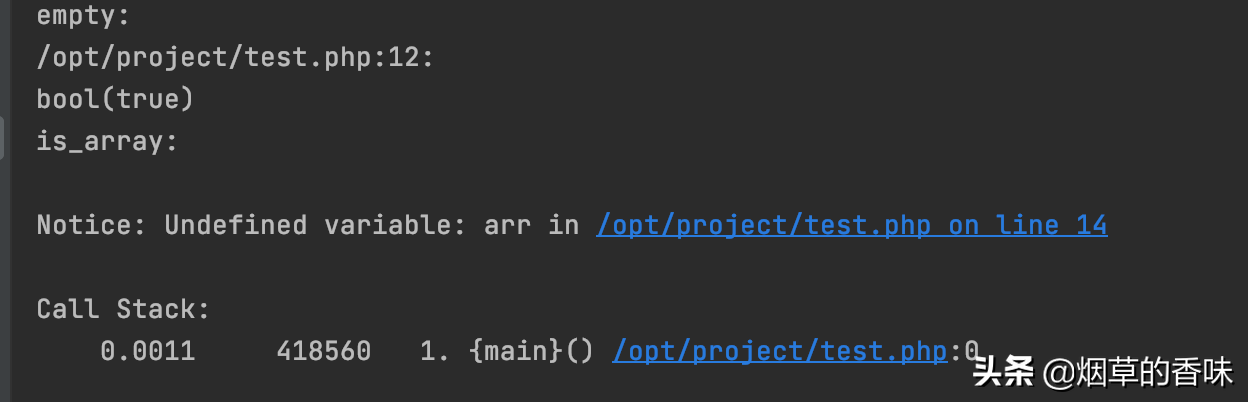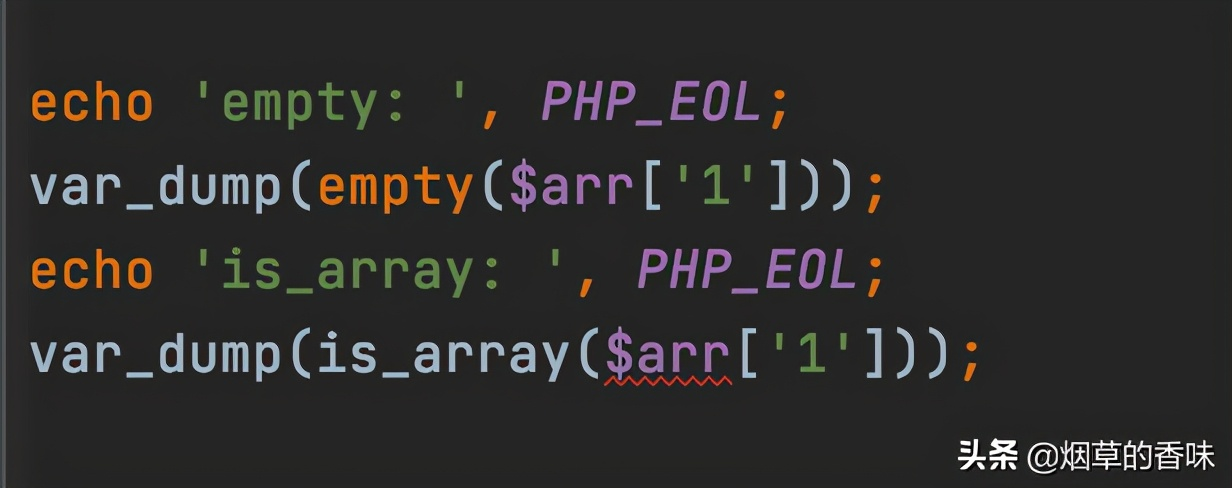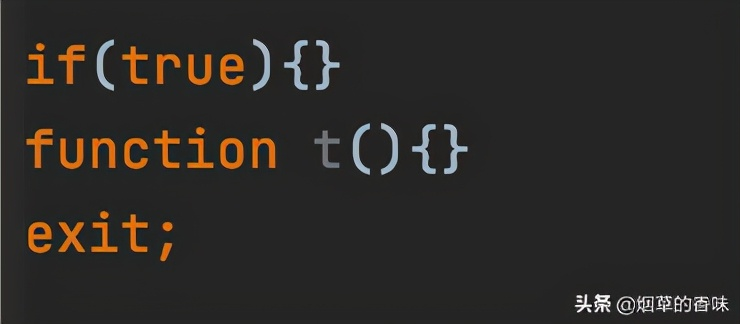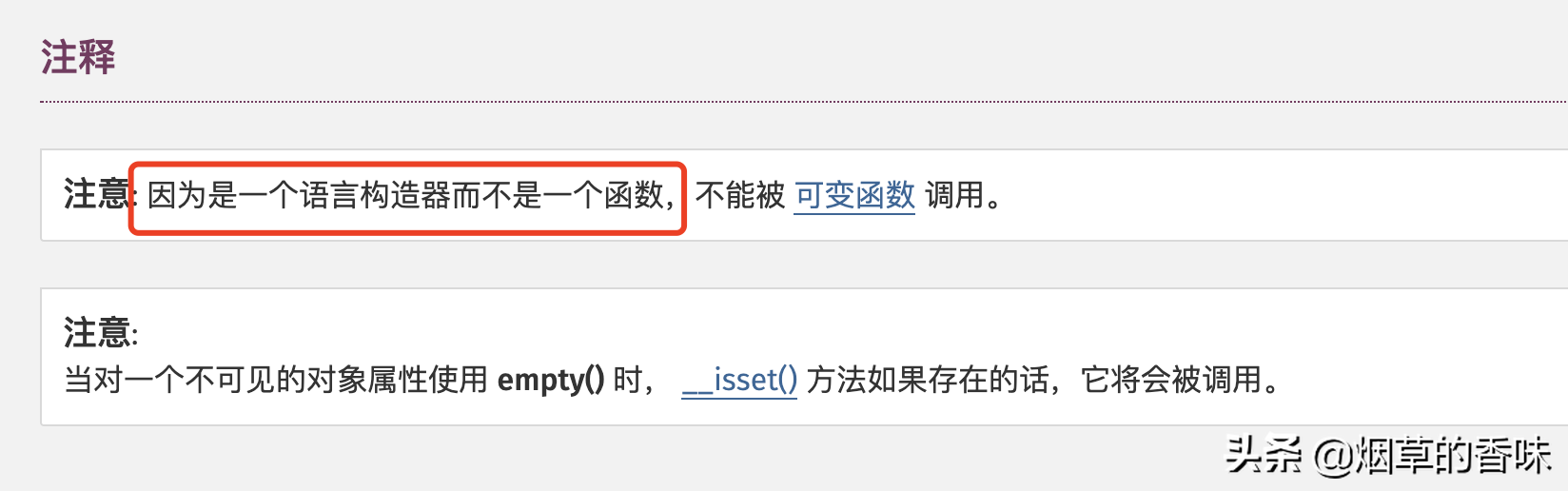# 语言构造器

``\$isArrFun = 'is_array'; var_dump(\$isArrFun(2)); \$empFun = 'empty'; var_dump(\$empFun(1));``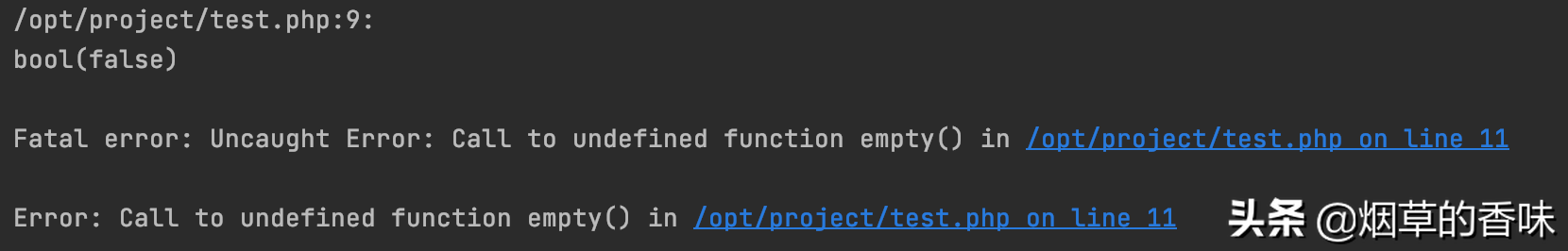``\$arr = [       'a' => null, ]; var_dump(isset(\$arr['a'])); var_dump(array_key_exists('a', \$arr));``